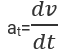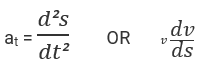# Tangential Velocity Formula

## Tangential Velocity Formulas - Derivation & Solved Examples

Tangential acceleration is the rate of change of velocity at a point in case of non-linear motion. It is always perpendicular to the centripetal acceleration at that point.
So, the rate of change of tangential velocity of at a point in a circular orbit is called Tangential acceleration.Where at = tangential acceleration
dv = tangential velocity
dt = change in time
Tangential acceleration in terms of displacement isWhere s = displacement
SI unit of tangential acceleration is m/s2

Example:
A body accelerates uniformly on a circular path with a speed of 10 m/s to 20m/s in 4s. Calculate its tangential acceleration.
Solution:
Given:
Initial velocity u = 10 m/s,
Final velocity v = 20 m/s,
Change in velocity dv = v – u = 20 – 10 = 10 m/s
Time taken dt = 4s
The tangential acceleration is given by at = dv / dt
= 10 / 4
= 2.5 m/s2.

Question:
A body accelerates uniformly at 2 m/s2. on a circular path with a speed of from rest. Find the speed in 4s.